# VBA CDECArticle byExcelMojo Team## What is Excel VBA CDec Function?

The VBA “CDEC” function is specifically used to convert expressions or values into Decimal data type. This data type can hold decimal numbers with high precision and a larger range of values than other numeric data types like Integer or Double.

Consider the following example. This example prints the product of three numbers mentioned inside a function in the Immediate tab.

###### Key Takeaways
• CDec is a built-in VBA function used to convert values to the Decimal data type. It is primarily used for precise decimal calculations, such as financial calculations or operations that require exact decimal representations.
• VBA CDec provides higher precision compared to other numeric data types like CDbl.
• It is useful when working with decimal values that require accurate representation, such as monetary amounts or tax calculations.
• The VBA CDec ensures that calculations involving decimal numbers are performed accurately, without the rounding errors that can occur with other data types.
• It is important to ensure that the values being passed to VBA CDec are within the valid range for the Decimal data type to avoid overflow errors.
• CDec can be used in various VBA applications, such as Excel, Word, or Access, for precise decimal calculations and formatting.

### VBA CDEC Syntax

The syntax of the CDec function is

CDec(Expression)

The “Expression” parameter represents the value or expression that you want to convert to the Decimal data type. It can be a numeric value, a variable, or a valid expression.

### How to Use the CDec Function in Excel VBA?

To implement the VBA CDec function, we perform the following steps, as shown below.

1. Go to the “Developer” section in the toolbar and click the “Visual Basic” option. Now, the VBA Editor opens to add functions and Sub procedures. Then, click the “Insert” and “Module” buttons to create a new module or blank page.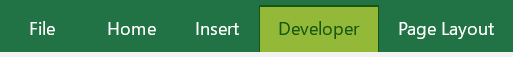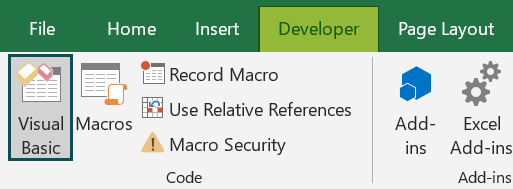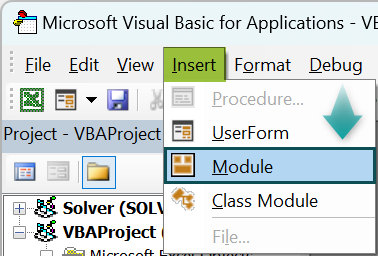2. Initialize a sub-procedure to convert a number to a decimal.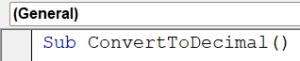3. Initialize a double variable, a variant datatype.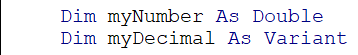4. Get a number from the user using the Inputbox function.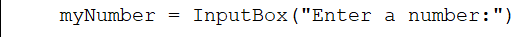5. Initialize an If-Else statement to check whether the given input is a number, then print the decimal value of it with the VBA CDec function.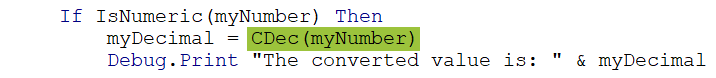6. It throws a VBA CDec type mismatch if it doesn’t clear it.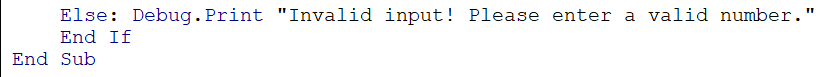Code:

Sub ConvertToDecimal()
Dim myNumber As Double
Dim myDecimal As Variant
myNumber = InputBox(“Enter a number:”)
If IsNumeric(myNumber) Then
myDecimal = CDec(myNumber)
Debug.Print “The converted value is: ” & myDecimal
Else: Debug.Print “Invalid input! Please enter a valid number.”
End If
End Sub

7. Print the decimal value printed in the code.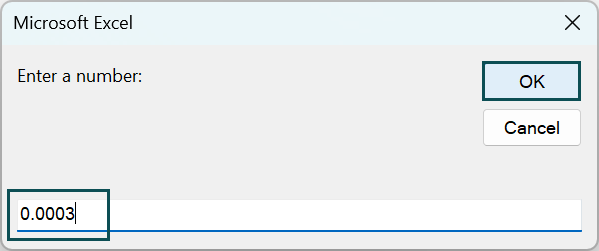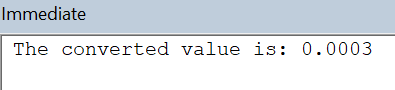For throwing VBA CDec type mismatch: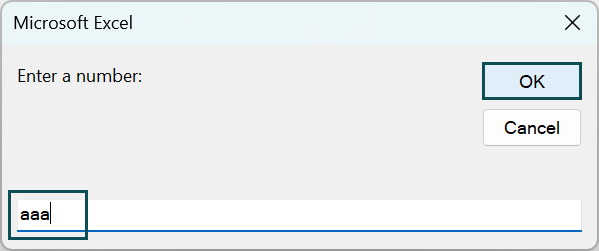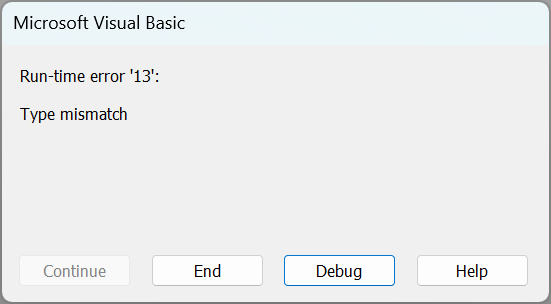### Examples of Using VBA CDEC Function

Let us look at examples of implementing the CDEC function in Excel VBA in suitable scenarios.

#### Example #1

Consider an example where we must calculate the total cost of a given quantity of a product with a user-defined cost.

• Step 1: Define a sub-procedure that prints the total cost using the VBA CDec function.
• Step 2: Declare quantity as an Integer, price as Currency datatype, and total as Currency datatype.
• Step 3: Input the quantity and price from the user.
• Step 4: Initialize an If-Else statement to check whether the price and quantity are integers. If they are valid numbers, the CInt function converts the quantity to the Integer data type, and the CDbl function converts the price to the Double data type. Next, the VBA code calculates the subtotal by multiplying the quantity and price.
• Step 5:  If they are valid numbers, the CInt function converts the quantity to the Integer data type, and the CCur function converts the price to the Currency data type.
• Step 6: The total price of the given quantities and cost is multiplied and stored in the total variable.
• Step 7: If the given numbers don’t satisfy the condition of integers, it throws a VBA CDec Error for data type mismatch.

Code:

Sub CalculateTotal()
Dim quantity As Integer
Dim price As Currency
Dim total As Currency
quantity = InputBox(“Enter the quantity:”)
price = InputBox(“Enter the price per unit:”)
If IsNumeric(quantity) And IsNumeric(price) Then
quantity = CInt(quantity)
price = CCur(price)
total = CDec(quantity) * price
Debug.Print “The total cost is: ” & total
Else
Debug.Print “Invalid input! Please enter valid numbers.”
End If
End Sub

• Step 8: Run the code for the above code. The output is shown below.

This prints the output in the Immediate tab.

#### Example #2

In this example, the code prompts the user to enter the quantity and price per unit using input boxes in VBA. The entered values are stored in the quantity and price variables, respectively.

• Step 1: Create a sub-routine to calculate sales tax.
• Step 2: Declare an Integer variable to accept a variable from the user as quantity, and the price, subtotal, taxRate as double, salesTax, and total As the Currency datatype.
• Step 3: Accept the quantity and price of the variable from the user.
• Step 4: Check whether the price and quantity are integers by initializing an If-Else statement.

If they are valid numbers, the CInt function is used to convert quantity to the Integer VBA data type, and the CDbl function is used to convert price to the Double data type. Next, the code calculates the subtotal by multiplying the quantity and price.

• Step 5: Accept the tax rate in percentage from the user and calculate the salesTax by multiplying the subtotal and taxRate.

The total sales are calculated by adding the CDec of subtotal value and salesTax.

• Step 6: After this, print all the values using the MsgBox function.

In VBA, vbCrLf is a predefined constant representing a carriage return (vbCr) followed by a line feed (vbLf), representing a new line or line break. The & operator is used for concatenation in VBA, allowing you to combine strings or values.

When vbCrLf is used with the & operator and an underscore (_) character, it is typically used to concatenate strings across multiple lines in a more readable format.

In this example, the MsgBox function displays a message box. The & operator is used to concatenate the values together.

Code:

Sub CalculateSalesTax()
Dim quantity As Integer
Dim price As Double
Dim subtotal As Double
Dim taxRate As Double
Dim salesTax As Currency
Dim total As Currency
quantity = InputBox(“Enter the quantity:”)
price = InputBox(“Enter the price per unit:”)
If IsNumeric(quantity) And IsNumeric(price) Then
quantity = CInt(quantity)
price = CDbl(price)
subtotal = quantity * price
taxRate = CDbl(InputBox(“Enter the tax rate (in percentage):”)) / 100
salesTax = CDec(subtotal * taxRate)
total = CDec(subtotal) + salesTax
MsgBox “Subtotal: ” & Format(subtotal, “#,##0.00”) & vbCrLf & _
“Tax Rate: ” & Format(taxRate, “0.00%”) & vbCrLf & _
“Sales Tax: ” & Format(salesTax, “#,##0.00”) & vbCrLf & _
“Total: ” & Format(total, “#,##0.00”)
Else
MsgBox “Invalid input! Please enter valid numbers.”
End If
End Sub

• Step 7: Print the above code. The output is printed in the MsgBox, by accepting all user inputs and calculating the total sales of quantity and products, including the sales tax.

Using the vbCrLf function, concatenate all the different values and display them in a single MsgBox, instead of printing them in different MsgBox popups.

### Important Things to Note

• Validate the input values and ensure they are within the valid range for the Decimal data type.
• Implement error handling techniques, such as the On Error statement, to handle potential VBA CDec overflow errors that may occur when using CDec with large values.
• Don’t ignore or suppress VBA CDec overflow errors, as they can lead to incorrect results or unexpected behavior in your VBA code.
• Use functions like IsNumeric or TryParse to validate the input before using CDec to avoid potential errors when dealing with VBA CDec Error.

1. What is type mismatch with cdec VBA in Excel?

A type mismatch with CDec in VBA occurs when the value passed to the CDec function is incompatible with the Decimal data type. It indicates that the value’s data type does not match the expected data type for conversion.

The VBA CDec type mismatch occurs when:

• The value being passed to CDec is not a numeric value: The CDec function expects a numeric value as input. If a non-numeric value, such as a string or an object, is provided, a type mismatch error will occur.

• The value being passed to CDec is incompatible: The input value should be in a format that can be interpreted as a number. For example, if you pass a string that cannot be interpreted as a valid numeric value, such as “abc” or “123abc”, a type mismatch error will occur.

• The value being passed to CDec is of a different numeric data type: While CDec is primarily used to convert values to the Decimal data type, if you pass a value that is already of a different numeric data type, such as Integer or Double, a type mismatch error may occur. In such cases, you may need to use a different conversion function appropriate for that data type, such as CInt or CDbl.

2. What is the difference between vba CDec vs CDbl?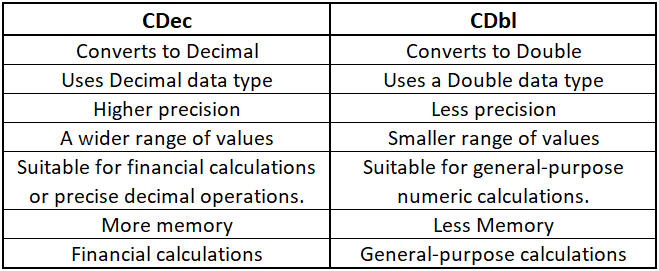3. Why is Excel VBA CDec Function not working?

• Invalid input: The value being passed to CDec might not be a valid number or in the correct format.
• Type mismatch: The data type of the value being passed to CDec might not be compatible with the Decimal data type.
Overflow: The value being converted might exceed the valid range for the Decimal data type, resulting in an overflow error.
• Missing reference: The reference to the library that includes the CDec function might be missing or not properly set.
• Localization issues: In some regional settings, the decimal separator used may be different, causing issues with the conversion.

This has been a guide to VBA CDEC. Here we learn how to use the CDec function in Excel VBA, its syntax & difference from CDbl, with step-by-step examples. You can learn more from the following articles –